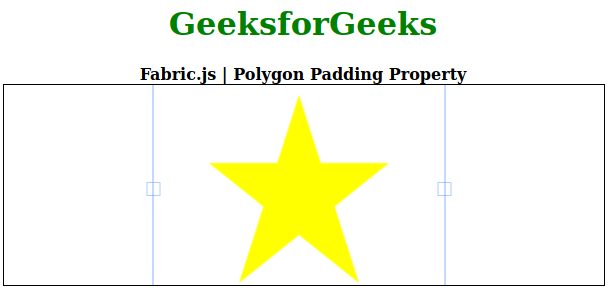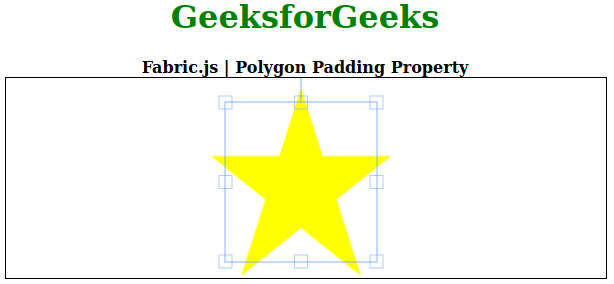• Last Updated : 26 Aug, 2020

In this article, we are going to see how to change the padding of a canvas polygon using FabricJS. The canvas polygon means the polygon is movable and can be stretched according to requirement. Further, the polygon can be customized when it comes to initial stroke color, height, width, fill color, or stroke width.

To make it possible we are going to use a JavaScript library called FabricJS. After importing the library, we will create a canvas block in the body tag that will contain the polygon. After this, we will initialize instances of Canvas and polygon provided by FabricJS and change the padding of the polygon using padding property and render the polygon on the Canvas as given in the example below.

Hey geek! The constant emerging technologies in the world of web development always keeps the excitement for this subject through the roof. But before you tackle the big projects, we suggest you start by learning the basics. Kickstart your web development journey by learning JS concepts with our JavaScript Course. Now at it's lowest price ever!

Syntax:

```fabric.Polygon([
{ x: pixel, y: pixel },
{ x: pixel, y: pixel },
{ x: pixel, y: pixel},
{ x: pixel, y: pixel},
{ x: pixel, y: pixel }],
{
});
```

Parameter: This property accepts a single parameter as mentioned above and described below:

• padding: It specifies the padding between the controlling border and the canvas object.

Note: Dimension pixels is must to create a polygon.

Below examples illustrate the Fabric.JS Polygon padding property in JavaScript:

Example 1: In this example, padding will be positive.

## HTML

 ` ``<``html``> `` ` `<``head``> ``    ````    ``<``script` `src``= ``"https://cdnjs.cloudflare.com/ajax/libs/fabric.js/3.6.2/fabric.min.js"``> ``    `` `` `` ` `<``body``> ``    ``<``div` `style``=``"text-align: center;width: 600px;"``> ``        ``<``h1` `style``=``"color: green;"``> ``            ``GeeksforGeeks ``        `` ``        ``<``b``> ``            ``Fabric.js | Polygon Padding Property ``        `` ``    `` `` ` `    ``<``canvas` `id``=``"canvas"``            ``width``=``"600"``            ``height``=``"200"``            ``style``=``"border:1px solid #000000;"``> ``    `` `` ` `    ``<``script``> `` ` `        ``// Initiate a Canvas instance ``        ``var canvas = new fabric.Canvas("canvas"); `` ` `        ``// Initiate a polygon instance ``        ``var polygon = new fabric.Polygon([ ``        ``{ x: 295, y: 10 }, ``        ``{ x: 235, y: 198 }, ``        ``{ x: 385, y: 78}, ``        ``{ x: 205, y: 78}, ``        ``{ x: 355, y: 198 }], { ``            ``fill: 'yellow',``            ``padding: 55``        ``}); `` ` `        ``// Render the polygon in canvas ``        ``canvas.add(polygon); ``    `` `` `` ` ``

Output:Example 2: In this example padding will negative.

## HTML

 ` ``<``html``> `` ` `<``head``> ``    ````    ``<``script` `src``= ``"https://cdnjs.cloudflare.com/ajax/libs/fabric.js/3.6.2/fabric.min.js"``> ``    `` `` `` ` `<``body``> ``    ``<``div` `style``=``"text-align: center;width: 600px;"``> ``        ``<``h1` `style``=``"color: green;"``> ``            ``GeeksforGeeks ``        `` ``        ``<``b``> ``            ``Fabric.js | Polygon Padding Property ``        `` ``    `` `` ` `    ``<``canvas` `id``=``"canvas"``            ``width``=``"600"``            ``height``=``"200"``            ``style``=``"border:1px solid #000000;"``> ``    `` `` ` `    ``<``script``> `` ` `        ``// Initiate a Canvas instance ``        ``var canvas = new fabric.Canvas("canvas"); `` ` `        ``// Initiate a polygon instance ``        ``var polygon = new fabric.Polygon([ ``        ``{ x: 295, y: 10 }, ``        ``{ x: 235, y: 198 }, ``        ``{ x: 385, y: 78}, ``        ``{ x: 205, y: 78}, ``        ``{ x: 355, y: 198 }], { ``            ``fill: 'yellow',``            ``padding: -15``        ``}); `` ` `        ``// Render the polygon in canvas ``        ``canvas.add(polygon); ``    `` `` `` ` ``

Output:My Personal Notes arrow_drop_up